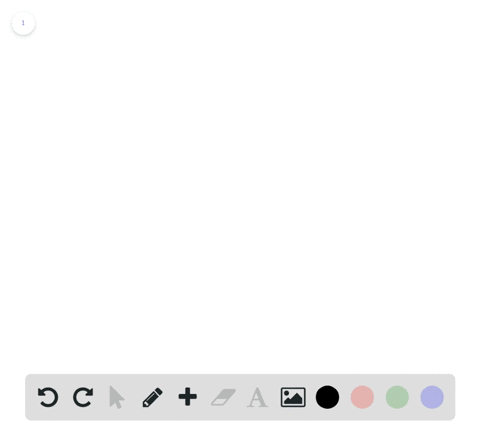🎉 The Study-to-Win Winning Ticket number has been announced! Go to your Tickets dashboard to see if you won! 🎉View Winning Ticket### Degrees to Radians In Exercises 9 and 10, convert…

01:04University of Southern California
Problem 8

# Coterminal Angles in Radians In Exercises 7 and 8 , determine two coterminal angles in radian measure (one positive and one negative) for each angle.

Check back soon!

## Discussion

You must be signed in to discuss.

## Video Transcript

access to determine to co terminal angles in radiance for each of the following angles. A positive co terminal angle and a negative co terminal angle. So one aspect of angles that we could leverage to help us solve this problem is that one full revolution from this horizontal line back to it is going to be to pie ingredients. So by adding or subtracting two pie every time we do that, that angle that we end up with is going to be co terminal. So for a if we take negative nine high fourths and add to high well, see, that is a co terminal angle. No, we can also express to pie as eight pi fourths, right? Just multiplying two in there. And that's gonna give us negative pie forth as a code terminal angle because we're asked to have one positive and one negative code terminal angle. I'm just gonna take negative pie fourths and then add eight pi forth once more and we're going to get seven pi fourths. So here's a positive code. Terminal angle and a negative paternal angle for party Now Part B were given a pine nineths as our starting angle, so What I'm gonna do is I'm gonna add to pie in the beginning. Okay? Another way that we can express to pie to make it easier to Abby's together would be 18 pi over nine. So eight pi over nine plus 18 pie overnight. All right, we're gonna add those together, and we see that 18 plus eight is 26. So we have 26 pie nights as our positive co terminal angle. Now, another direction we can go is to take a pine nights and then subtract to pie. And that's the same is writing a pie nineths minus 18. Pie nineths equals while eight minus 18 is negative. 10. So negative 10 hi nights. So this is a positive and a negative co terminal angle for the two angles listed here in A and B